Created May 20, 2018

# New Senses for Buildings

Sensors & predictive analytics solution to save energy & reduce costs of high value air distribution systems.

65

## Things used in this project

### Hardware componentsArduino Nano R3
×1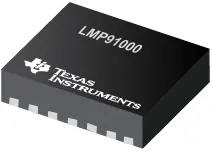Texas Instruments Gas / Chemical Sensors
×1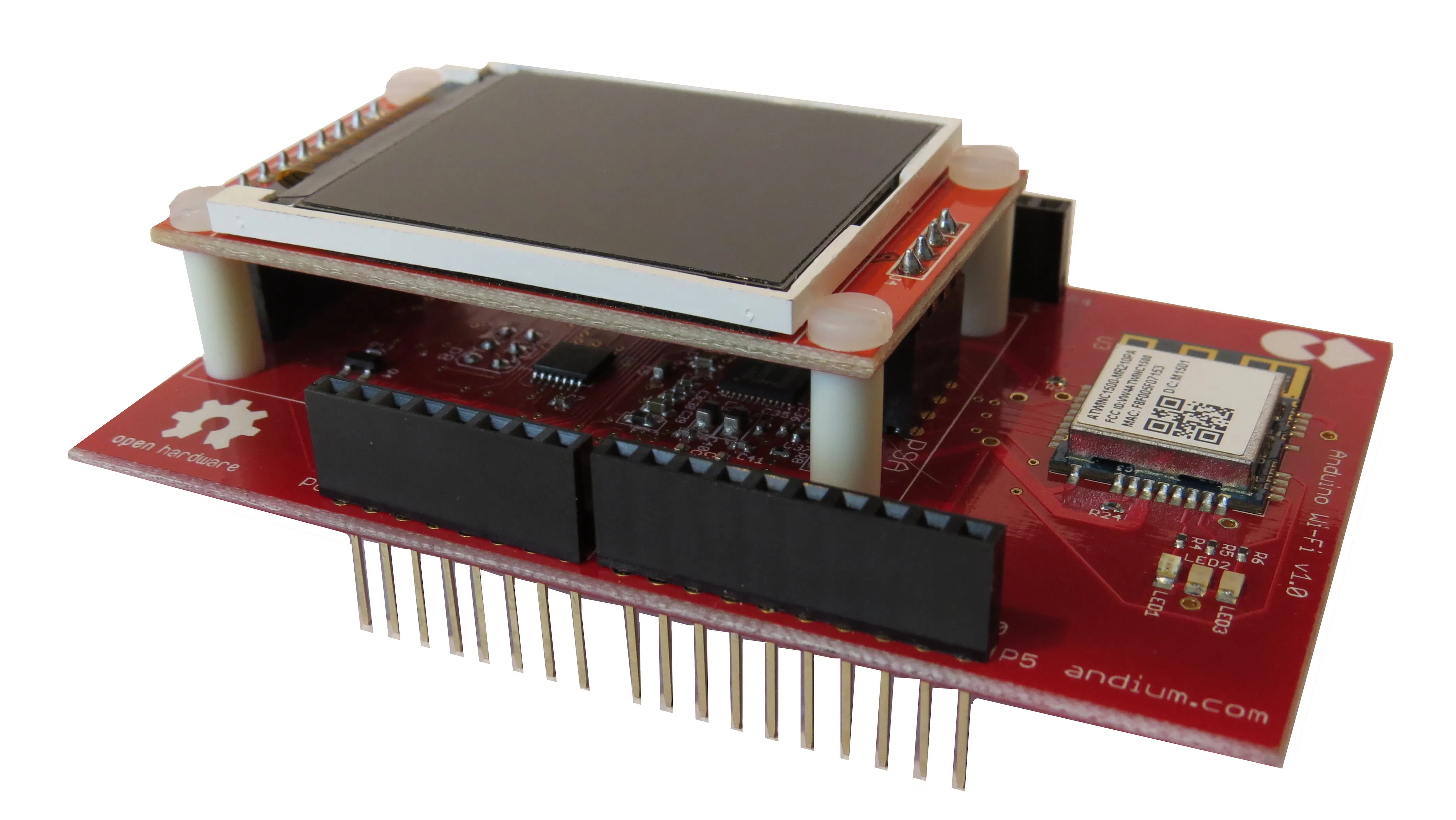Andium Anduino WiFi
×1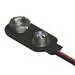9V Battery Clip
×1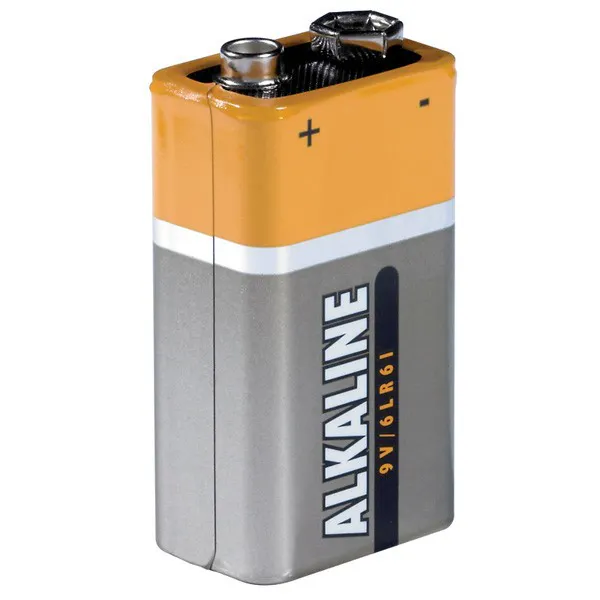9V battery (generic)
×2

### Hand tools and fabrication machines3D Printer (generic)Soldering iron (generic)

## Schematics

### Sensor image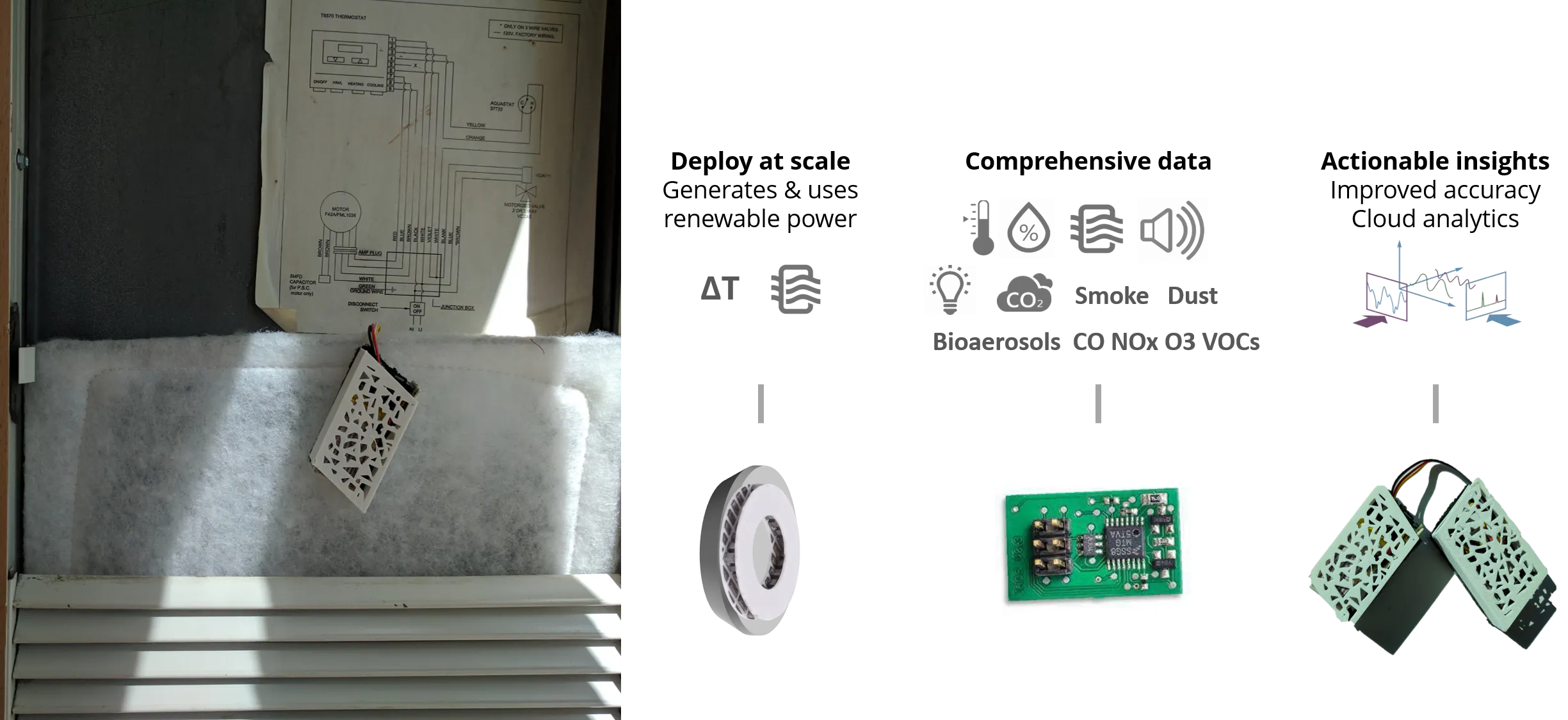### Schematic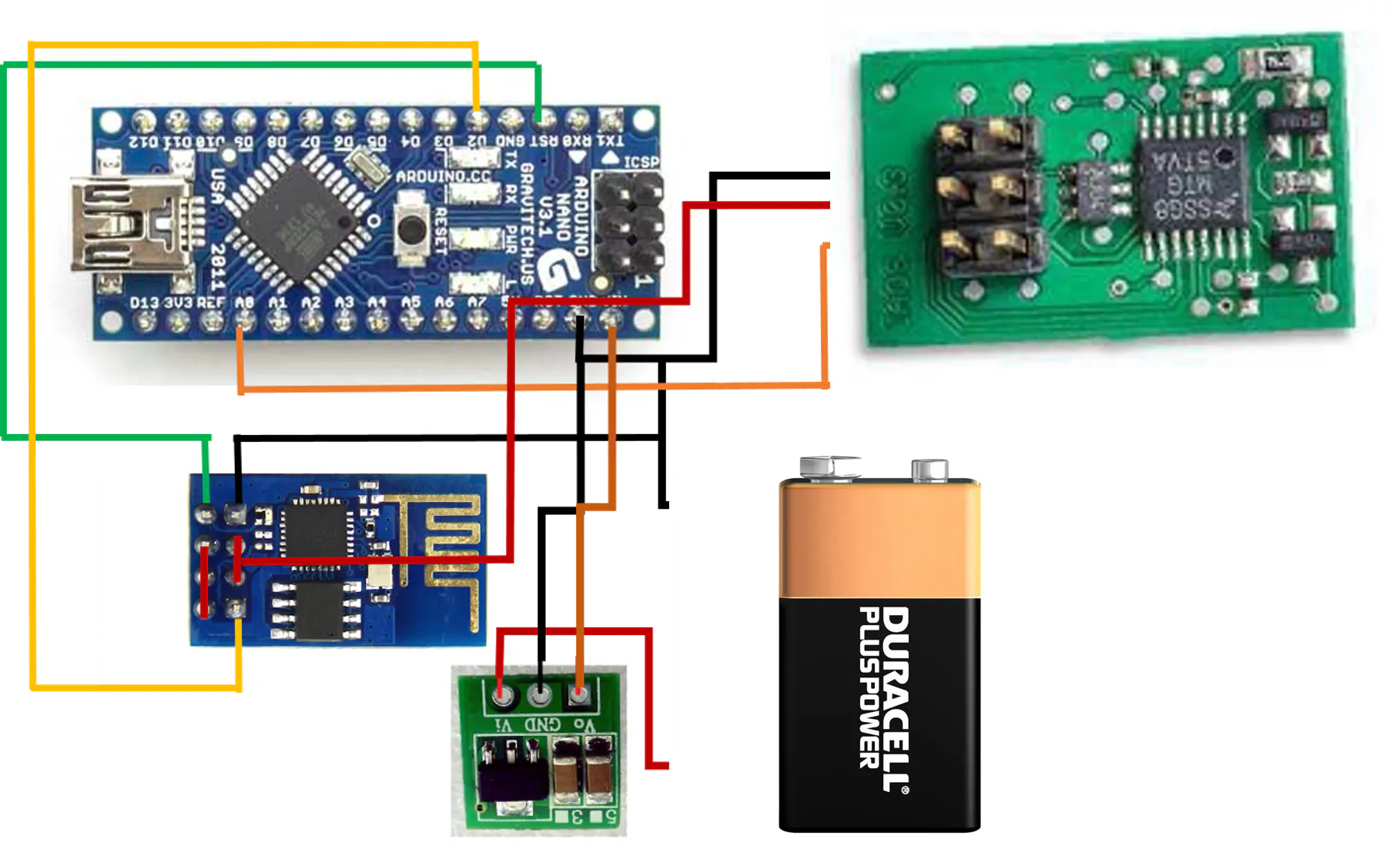## Code

### Sensor Code

C/C++
```#include <Wire.h> // Wire library that helps the Arduino with i2c
#include <Streaming.h> // Streaming C++-style Output with Operator << from http://arduiniana.org/libraries/streaming/
#include <String.h>

//------------------------------------------------------------------------------
// CONFIGURATION
//------------------------------------------------------------------------------

// define FAST sensor sampling interval
unsigned long INTERVAL_FAST = 5000; // sample duration (ms)30s, david holstius used 60000
unsigned long last_time_fast_ms;     // time of last check/output

String s3 = "C7=";
String s = "";
String si2="";
String sd="SF";
//int x=0;

//wifi global variables
int OK = 0; // this tells me if the data was received
int SIGNAL = 0; // this tells me if I get a response which indicates that there is communcition between module and server
int stat = 0;

// SD card variables
String network; // this is the wifi network name that is specified in the conf.txt file
String password; // this is the wifi network password that is specified in the conf.txt file
char filename[] = "conf.txt"; // this is the name of the file that holds the wifi info

int sdc=0;
int r=0;

//------------------------------------------------------------------------------
// COMPONENT SETUP
//------------------------------------------------------------------------------

//-------------------------------------------------------------------------- MUX

int MUX_BASE = 0; // analog pin to interact with mux
int MUX_CONTROL[] = {
2, 3, 4, 5}; // digital pins for mux pin selection
int mux(int channel) {
// select channel on multiplexer
for (int i = 0; i < 4; i++) {
}
// return base pin for analogRead/Write to use
return(MUX_BASE);
}

void setup_mux() {
// set digital pins controlling multiplexer to OUTPUT
for (int i = 0; i < 4; i++) {
pinMode(MUX_CONTROL[i], OUTPUT);
}
}

//------------------------------------------------------------ SD card (logging)

#include "SD.h" //SD library to talk to the card
#define SD_PIN 10 // for the data logging shield, we use digital pin 10 for the SD cs line
#define SD_INTERVAL_SYNC 1000 // mills between calls to flush() - to write data to the card
uint32_t sd_last_time = 0; // time of last sync()
File logfile; // the logging file
File myFile;

void GetCredentials()
{
char index1[] = "network:"; // this is used to make sure that the network name is right after the label "network:"
char index2[] = "password:"; // this is used to make sure that the password is right after the label "password:"
int i1 = 0; // this is used to match the character coming from the serial with the character in the "network"
int i2 = 0; // this is used to match the character coming from the serial with the character in the "password"

pinMode(SD_PIN, OUTPUT);
Serial.println("initializing");

if (!SD.begin(SD_PIN)) { // if the SD card did not begin it will send a warning
Serial.println("initialization failed");
return;
}

Serial.println("initialization began");

if (SD.exists(filename)) {
myFile = SD.open(filename); // open the file

if (myFile) {
int stop1 = 0; // this is used to indicate when the network name is done recording and the password can begin to be recorded

while (myFile.available()) {

if (u!=index1[i1] && stop1 == 0 && u != '\n') { network = network+u; } // record the network name
if (u==index1[i1] && stop1 == 0) { i1++; }
if (u=='\n') { stop1 = 1;}

if (u!=index2[i2] && stop1 == 1 && u!='\n') { password = password + u; } // record the password
if (u==index2[i2] && stop1 == 1) { i2++; }

}
myFile.close();
} else { Serial.println("error opening"); }
}
}

// setup
void setup_sd() {

// initialize the SD card and find a FAT16/FAT32 partition
//  log_info("SDcard", "initializing...");

//digitalWrite(SD_PIN, HIGH);
pinMode(SD_PIN, OUTPUT); // default chip select pin is set to output

// create a new file, LOGGERnnnn.csv (nnnn is a number), make a new file every time the Arduino starts up
char filename[] = "0000.csv";
for (uint16_t i = 0; i < 10000; i++) {

for (int j = 0; j < 4; j++) {
filename[4-j-1] = ((int)(i / pow(10, j)) % 10) + '0';
}

if(!SD.exists(filename)) { // only open a new file if it doesn't exist
logfile = SD.open(filename, FILE_WRITE); // Unix style command flags - logfile.open() procedure FILE_WRITE - create the file and write data to it
break;  // leave the loop!
}
}
if (!logfile) {
//logfile<<"could not create file"<<endl;
sdc=1;
}

sd_last_time = millis();
}

// --------------------------------------------------- WiFi setup

/* this is the function for connecting to the wifi network, I need to know the network name and password before i can connect */

void setupWIFI()
{

OK=0;
while (OK==0) // this will keep trying to connect until it has received an "OK" from the modem
{
Serial.println("AT+RST");
delay(10000);

Serial.println("AT+CIPSTATUS");
if (Status() == 2) break;

//Serial.println("AT+CWJAP=\"" + String(network) + "\",\"" + String(password) + "\"");
Serial.println("AT+CWJAP=\"SOCAAR\",\"Particle\$f1yf@r\"");
delay(15000);

Serial.println("AT+CIPSTATUS");
if (Status() == 2) break;

}

Serial.println("AT+CIFSR");
delay(1000);
//ShowSerialData();

Serial.println("AT+CIPMUX=0");
delay(2000);
//ShowSerialData();
}

//------------------------------------------------------ Chronodot (timekeeping)

#include "Chronodot.h" // Chronodot RTC library
Chronodot RTC;

// setup
void setup_rtc() {
// kick off the RTC initializing the Wire library - poking the RTC to see if its alive
Wire.begin();
RTC.begin(); // Chronodot
}

void log_time2() {
DateTime now = RTC.now();
int year  = now.year();
int month = now.month();
int day   = now.day();
int hours = now.hour();
int mins  = now.minute();
int secs  = now.second();
logfile << "\"" << year;
logfile << "-" << ((month<10)?"0":"") << month;
logfile << "-" << ((day<10)?"0":"")   << day;
logfile << " " << ((hours<10)?"0":"") << hours;
logfile << ":" << ((mins<10)?"0":"")  << mins;
logfile << ":" << ((secs<10)?"0":"")  << secs;
logfile << "\",";
}

//---------------------------------------------------------- DHT22 (temperature)

#include "DHT.h"
#define DHT_PIN 7
#define DHT_TYPE DHT22   // DHT 22  (AM2302)
DHT dht(DHT_PIN, DHT_TYPE);

// setup
void setup_dht() {
dht.begin();
}

// log
void log_dht() {
// Sensor readings may also be up to 2 seconds 'old' (its a very slow sensor)
// check if returns are valid, if they are NaN (not a number) then something went wrong!
if (isnan(t) || isnan(h)) {
}
else {
logfile<<h<<",";
logfile<<t<<",";

}
s+=String(h)+",";
s+=String(t)+",";
}

//----------------------------------------------------------- Sinyei (particles)

#define SNY_PIN 8
unsigned long sny_duration;
unsigned long sny_lowocc_us = 0; // sum of time spent LOW

unsigned long SNY_INTERVAL_ms = 30000; // sample duration (ms)30s, david holstius used 60000
unsigned long sny_last_time;           // time of last serial dump

// setup
void setup_sinyei() {
pinMode(SNY_PIN, INPUT);
sny_last_time = millis();
sny_lowocc_us = 0;
}

void log_sinyei(unsigned long lowocc_us, unsigned long sampletime_ms) {
float ratio; // fraction of time spent LOW

ratio = lowocc_us / (sampletime_ms * 1000.0) * 100.0;
logfile<<ratio<<",";

si2=String(ratio);
}

//------------------------------------------------------------ Sharp (particles)

// connected to analog 1 and controlled by digital 9
#define SRP_PIN_DUST 1
#define SRP_PIN_LED 9 // led Power is any digital pin on the arduino connected to Pin 3 on the sensor (can be 2, 4,)

int srp_delay1_us = 280; // delays are in microseconds
int srp_delay2_us = 40;
int srp_delayoff_us = 9680;

// setup
void setup_sharp() {
pinMode(SRP_PIN_LED, OUTPUT);
}

// log
void log_sharp() {
digitalWrite(SRP_PIN_LED, LOW); // power on the LED
delayMicroseconds(srp_delay1_us);
delayMicroseconds(srp_delay2_us);
digitalWrite(SRP_PIN_LED, HIGH); // turn the LED off
delayMicroseconds(srp_delayoff_us);
//
logfile<<dustVal<<",";
s+=String(dustVal);
}

//------------------------------------------------------------------------------
// SETUP
//------------------------------------------------------------------------------

void(* resetFunc) (void) =0;

void setup(void) {

// setup serial
Serial.begin(115200);

delay(5000);

delay(500);
setupWIFI();

setup_rtc(); // all logging depends on RTC, so start it up first
setup_sd();
setup_mux();
setup_dht();
setup_sinyei();
setup_sharp();

// set fast sensor clock
last_time_fast_ms = millis(); // get the current time

s = s3;

}

//------------------------------------------------------------------------------
// MAIN LOOP
//------------------------------------------------------------------------------

void loop() {

logger();

s+=","+si2;

if (sdc==1) {
s+=","+sd;
sdc=0;
}

si2="";
sendtoExosite();
s=s3;

// reset clock to current time
last_time_fast_ms = millis();

//--------------------------------------------------------------- sync SD card

// write data to disk! Don't sync too often (2048 bytes of I/O SD card, a lot of power, takes time)
if ((millis() - sd_last_time) > SD_INTERVAL_SYNC) {
logfile.flush();
sd_last_time = millis();
}

}

void logger() {

sny_duration = pulseIn(SNY_PIN, LOW);
sny_lowocc_us += sny_duration;

//-------------------------------------- read/lMog Sinyei sensor (30s interval)

if ((millis() - sny_last_time) > SNY_INTERVAL_ms) {

// log Sinyei
log_sinyei(sny_lowocc_us, millis() - sny_last_time);
// reset
sny_lowocc_us = 0;
sny_last_time = millis();

}

logfile<<endl;

//log_time2();
//log_dht();
//log_sharp();

int num_sensors = 16;
int sensor_value;

for (int channel = 0; channel < num_sensors; channel++) {
if (channel==0 || channel==1 || channel==2 || channel==4 || channel==5 || channel==6 || channel==7 || channel==9 || channel==10 || channel==11){
logfile<<sensor_value<<",";
s+=",";
s+=String(sensor_value);
}
}
delay(100);
}

void sendtoExosite()
{

int num;
int num2;
String le;
String le2;
num=s.length();
num2= num+204;

le=String(num);
le2=String(num2);

Serial.println("AT+CIPSTART=\"TCP\",\"m2.exosite.com\",80");
delay(1000);

Serial.println("AT+CIPSEND="+le2);
delay(200);
Serial.flush();

Serial.print("POST /api:v1/stack/alias HTTP/1.1\r\n");
Serial.print("Host: m2.exosite.com\r\n");
Serial.print("X-Exosite-CIK: 1e6012249cd51557a55b6ec8305543a53ec93d83\r\n");
Serial.print("Content-Type: application/x-www-form-urlencoded\r\n");
Serial.print("Content-Length: "+le+"\r\n\r\n");
Serial.print(s+"\r\n\r\n");
delay(500);

Serial.flush();
delay(500);
ShowSerialData();

Serial.println((char)26);

Serial.println("AT+CIPCLOSE");
delay(2500);

if (OK==0) //if the response did not read 'OK' then that means that the data did not send and is probably not connected so I have to reconnect
{
Serial.println("NOT WORKING");
setupWIFI();
}
}

void ShowSerialData() // this is where I view the response and index OK if I am using software serial or just index OK if i am using hardware serial
{
OK = 0;
int i1 = 0;
int i2 = 0;
char inChar;
while(Serial.available()!=0)
{
//Serial.write(inChar);
if (inChar=='1') {
SIGNAL++;
}
if (inChar=='O') {
i2=i1;
}
if (inChar=='K' && i1-i2==1) {
OK++;
}
i1++;
}
}

int Status()
{
char inChar;
stat=0;
while (Serial.available()!=0)
{
//Serial.write(inChar);
if (inChar=='2') stat = 2;
}
return stat;
}
```

## Credits

### Lia

2 projects • 0 followers